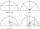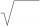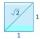# Logs

Trunk diameter is 52 cm. Is it possible to inscribe a square prism with side 36 cm?

Result

#### Solution:We would be pleased if you find an error in the word problem, spelling mistakes, or inaccuracies and send it to us. Thank you!Tips to related online calculators
Do you want to convert length units?
Pythagorean theorem is the base for the right triangle calculator.

#### You need to know the following knowledge to solve this word math problem:

We encourage you to watch this tutorial video on this math problem:

## Next similar math problems:

• Numeric questionIt is possible negative irrational number?
• RootsCalculate the square root of these numbers:
• Transforming cuboidCuboid with dimensions 6 cm, 10, and 11 cm is converted into a cube with the same volume. What is its edge length?
• Expression 6Evaluate expression: -6-2(4-8)-9
• NumberIs number 5.146852 irrational?